Publication date: 11/29/2021

##Random Coefficient Model

The standard random coefficient model specifies a random intercept and slope for each subject. Let yij denote the measurement of the jth observation on the ith subject. Then the random coefficient model can be specified as follows: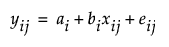where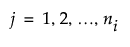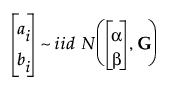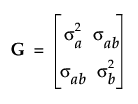and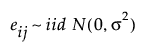You can reformulate the model to reflect the fixed and random components that are estimated: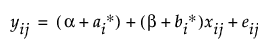where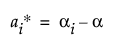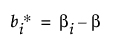and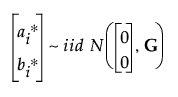with G and eij defined as above.